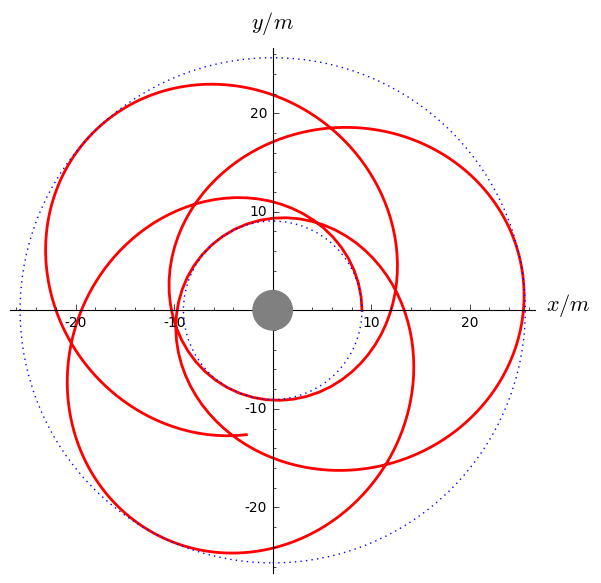# Timelike orbits in Schwarzschild spacetime¶

This Jupyter/SageMath worksheet is relative to the lectures Geometry and physics of black holes.

Click here to download the worksheet file (ipynb format). To run it, you must start SageMath with the Jupyter notebook, with the command sage -n jupyter

It uses the integrated_geodesic functionality introduced by Karim Van Aelst in SageMath 8.1, in the framework of the SageManifolds project.

A version of SageMath at least equal to 8.1 is required to run this worksheet:

In :
version()

Out:
'SageMath version 8.1, Release Date: 2017-12-07'
In :
%display latex  # LaTeX rendering turned on


We define first the spacetime manifold $M$ and the standard Schwarzschild-Droste coordinates on it:

In :
M = Manifold(4, 'M')
X.<t,r,th,ph> = M.chart(r't r:(0,+oo) th:(0,pi):\theta ph:\phi')
X

Out:

For graphical purposes, we introduce $\mathbb{R}^3$ and some coordinate map $M\to \mathbb{R}^3$:

In :
R3 = Manifold(3, 'R^3', latex_name=r'\mathbb{R}^3')
X3.<x,y,z> = R3.chart()
to_R3 = M.diff_map(R3, {(X, X3): [r*sin(th)*cos(ph),
r*sin(th)*sin(ph), r*cos(th)]})
to_R3.display()

Out:

Next, we define the Schwarzschild metric:

In :
g = M.lorentzian_metric('g')
m = var('m'); assume(m >= 0)
g[0,0], g[1,1] = -(1-2*m/r), 1/(1-2*m/r)
g[2,2], g[3,3] = r^2, (r*sin(th))^2
g.display()

Out:

We set the specific conserved energy and angular momentum:

In :
#var('E L', domain='real')
E = 0.973
L = 4.2*m

In :
u = M.vector_field(name='u')
u = E/(1-2*m/r)
u = sqrt(E^2 - (1-2*m/r)*(1+L^2/r^2))
u = L/(r^2*sin(th)^2)
u.display()

Out:
In :
g(u,u).display()

Out:

Pericenter and apocenter:

In :
eq = (r^3*((1-2/r)*(1+L^2/m^2/r^2) - E^2)).simplify_full()
eq

Out:
In :
R.<w> = RR[]
print(R)

Univariate Polynomial Ring in w over Real Field with 53 bits of precision

In :
eqp = R(eq.subs(r=w))
eqp

Out:
In :
roots = sorted(eqp.real_roots())
roots

Out:
In :
r_per = roots*m
r_apo = roots*m
r_per, r_apo

Out:

We pick an initial point and an initial tangent vector:

In :
r0 = r_per
p0 = M.point((0, r0, pi/2, 1e-12), name='p_0')
Tp0 = M.tangent_space(p0)
u0 = u.at(p0)
u0.set_name('u_0')
print(u0)

Tangent vector u_0 at Point p_0 on the 4-dimensional differentiable manifold M

In :
u0.display()

Out:

Let us check that the scalar square of $u_0$ is $-1$, by means of the metric tensor at $p_0$:

In :
g0 = g.at(p0)
print(g0)

Symmetric bilinear form  g on the Tangent space at Point p_0 on the 4-dimensional differentiable manifold M


The scalar square is indeed equal to $-1$:

In :
g0(u0,u0).simplify_full()

Out:

### Check¶

Let us compute the conserved energy and angular momentum along the geodesic by taking scalar products of the 4-velocity $v_0$ with the Killing vectors $\xi=\frac{\partial}{\partial t}$ and $\eta=\frac{\partial}{\partial t}$:

In :
xi = X.frame()
eta = X.frame()
xi, eta

Out:
In :
xi0 = xi.at(p0)
eta0 = eta.at(p0)
print(xi0)
print(eta0)

Tangent vector d/dt at Point p_0 on the 4-dimensional differentiable manifold M
Tangent vector d/dph at Point p_0 on the 4-dimensional differentiable manifold M


The specific conserved energy $\varepsilon$ is:

In :
- g0(xi0, u0)

Out:

while the specific conserved angular momentum $\ell$ is

In :
g0(eta0, u0)

Out:

We declare the geodesic through $p_0$ having $v_0$ as inital vector, denoting by $s$ the affine parameter (proper time):

In :
s = var('s')
geod = M.integrated_geodesic(g, (s, 0, 1500), u0); geod

Out:

We ask for the numerical integration of the geodesic, providing some numerical value for the parameter $m$, and then plot it in terms of the Cartesian chart:

In :
sol = geod.solve(step=4, parameters_values={m: 1})  # numerical integration
interp = geod.interpolate()                 # interpolation of the solution for the plot

In :
graph = geod.plot_integrated(chart=X3, mapping=to_R3, ambient_coords=(x,y), plot_points=500,
thickness=2, label_axes=False)

In :
graph += circle((0,0), r_per/m, linestyle=':')
graph += circle((0,0), r_apo/m, linestyle=':')
graph += circle((0,0), 2, fill=True, edgecolor='grey', facecolor='grey')
show(graph, aspect_ratio=1, axes_labels=[r'$x/m$', r'$y/m$'])In :
file_name = "ges_orbit_e{:.3f}_l{:.3f}.pdf".format(float(E), float(L/m))
graph.save(file_name, aspect_ratio=1, axes_labels=[r'$x/m$', r'$y/m$'])
file_name

Out:

Some details about the system solved to get the geodesic:

In :
geod.system(verbose=True)

Geodesic in the 4-dimensional differentiable manifold M equipped with Lorentzian metric g on the 4-dimensional differentiable manifold M, and integrated over the Real interval (0, 1500) as a solution to the following geodesic equations, written with respect to Chart (M, (t, r, th, ph)):

Initial point: Point p_0 on the 4-dimensional differentiable manifold M with coordinates [0, 9.05774475732293*m, 1/2*pi, 1.00000000000000e-12] with respect to Chart (M, (t, r, th, ph))
Initial tangent vector: Tangent vector u_0 at Point p_0 on the 4-dimensional differentiable manifold M with components [1.248725471366881, 0, 0, 0.0511928294380894/m] with respect to Chart (M, (t, r, th, ph))

d(t)/ds = Dt
d(r)/ds = Dr
d(th)/ds = Dth
d(ph)/ds = Dph
d(Dt)/ds = 2*Dr*Dt*m/(2*m*r - r^2)
d(Dr)/ds = -(4*Dth^2*m^2*r^3 - 4*Dth^2*m*r^4 + Dth^2*r^5 - 4*Dt^2*m^3 + 4*Dt^2*m^2*r + (Dr^2 - Dt^2)*m*r^2 + (4*Dph^2*m^2*r^3 - 4*Dph^2*m*r^4 + Dph^2*r^5)*sin(th)^2)/(2*m*r^3 - r^4)
d(Dth)/ds = (Dph^2*r*cos(th)*sin(th) - 2*Dr*Dth)/r
d(Dph)/ds = -2*(Dph*Dth*r*cos(th) + Dph*Dr*sin(th))/(r*sin(th))


Out:

We recognize in the above list the Christoffel symbols of the metric $g$:

In :
g.christoffel_symbols_display()

Out:
In [ ]: